# The magnetic helicity

The magnetic helicity is the average linking number over all pairs of flux tubes, weighted by linking number.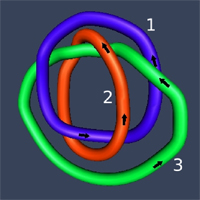A magnetic field consisting of three flux tubes. The linking numbers are L12=-1, L13=-1, and L23=1.

For example, consider a magnetic field which vanishes everywhere except in three flux tubes, of fluxes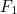,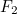and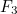, as shown in the figure. We calculate the linking numbers between each pair of tubes, which we denote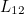,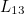, and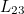. Then the magnetic helicity is given by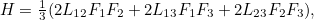where the factors of 2 are included by convention. If all three tubes had flux 1 (say), then the linkage in figure would give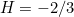. On the other hand, changingto 2 would change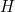to -2. Someasures both the type of linking and the (average) amount of magnetic flux that is linked. Of course, a real magnetic field will fill the whole of space with numerous flux tubes of varying fluxes. But we calculatein just the same way, averaging over all possible pairs of tubes. (Technically, larger flux tubes must first be split into multiple smaller flux tubes, so as to count the linking of different bundles of field lines within the larger tube.)

Back to main article

• Want facts and want them fast? Our Maths in a minute series explores key mathematical concepts in just a few words.